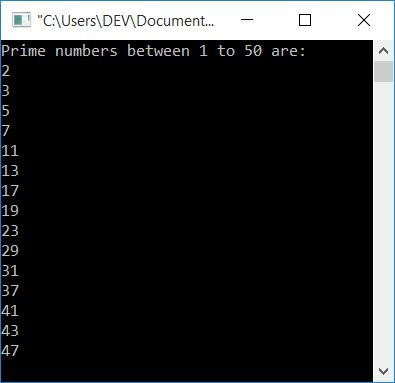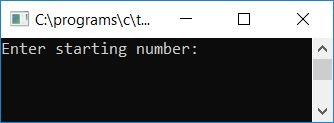# C Program to Print Prime Numbers

In this tutorial, you will learn and get code about the printing of prime numbers in the following ways:

But before going through the program, let's first understand prime numbers.

### What is a prime number?

A prime number is a number that can only be divisible by 1 and the number itself. That is, if a number is not divisible by anything except 1 and the number itself, then it is called a prime number. For example, 13, 23, and 37 are prime numbers, because 13 is not divisible by anything except 1 and 13. Like this, 23 is also not divisible by anything except 1 and 23.

## Print prime numbers from 1 to 50

The question is, "Write a program in C to print all prime numbers from 1 to 50." Here is its answer:

```#include<stdio.h>
#include<conio.h>
int main()
{
int i, count=0, j;
printf("Prime numbers between 1 to 50 are:\n");
for(i=1; i<=50; i++)
{
for(j=2; j<i; j++)
{
if(i%j==0)
{
count++;
break;
}
}
if(count==0 && i!=1)
printf("%d\n", i);
count = 0;
}
getch();
return 0;
}```

As the program was written in the Code::Blocks IDE, here is the sample run after a successful build and run:#### Program Explained

• Take a variable, say count, and initialize it with 0 at the beginning of the program.
• Create a for loop and start it from 1 to 50.
• Inside the for loop, create another for loop with a different loop variable, say j.
• Start the inner for loop with 2 and run up to one less than the value of the outer for loop's variable, say i.
• Then inside the second for loop, create an if block to check whether the number is divisible by any number from 2 to one less than the number. That is, if the number is 17, determine whether or not it is divisible by any of the numbers 2, 3, 4,... 15, 16.
• If it is, then increment the value of count and use the break statement to exit from the inner for loop.
• Otherwise, continue checking divisibility up to one less than the number. That is, if the number is 17, then check up to 16, exit from the loop, and check for the next number, say 18.
• On exiting from the inner for loop with or without using a break statement
• Check whether the variable count holds its original value (that is, 0) or not.
• If it holds, then the number is not divisible by anything; it means that the number is a prime number.
• And if the number is a prime number, then print the number as output and continue.
• If it does not hold its original value (that is, 0), then the number is divisible by something, which means that the number is not a prime number.
• Never forget to initialize 0 to count at the end of the first for loop.

## Print Prime Numbers Within a Specific Range

Now let's modify the above program by adding some extra features. In this program, we've added a feature that allows the user to specify the range in which all prime numbers should be printed. That is, this program will ask the user to enter a starting number of 10 and an ending number of 100 to print all the prime numbers present between these two numbers, 10 and 100, as shown in the program given below:

```#include<stdio.h>
#include<conio.h>
int main()
{
int i, count=0, j, start, end;
printf("Enter starting number: ");
scanf("%d", &start);
printf("Enter ending number: ");
scanf("%d", &end);
printf("\nPrime numbers between %d to %d are:\n", start, end);
for(i=start; i<=end; i++)
{
for(j=2; j<i; j++)
{
if(i%j==0)
{
count++;
break;
}
}
if(count==0 && i!=1)
printf("%d ", i);
count = 0;
}
getch();
return 0;
}```

Here is the first snapshot of the sample run:Now supply any number, say 10 as the starting number and 100 as the ending number, to print all the prime numbers present between these two numbers as shown in the second snapshot of the sample run:#### The same program in different languages

C Quiz

« Previous Program Next Program »